Science Innovation
Volume 3, Issue 6, December 2015, Pages: 63-67

Chain Rule for Fractional Order Derivatives

Ali Karci

Department of Computer Engineering, Faculty of Engineering, İnönü University, Malatya, Turkey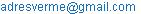Ali Karci. Chain Rule for Fractional Order Derivatives.Science Innovation.Vol.3, No. 6, 2015, pp. 63-67. doi: 10.11648/j.si.20150306.11

Abstract: The concept of derivative is an old concept and there are numerous studies on this concept. Some of these studies are on fractional order derivative. In this paper, we will emphasize that the methods for fractional order derivative are not valid for chain rule, and all definitions for fractional order derivatives have some deficiencies, since the basic concepts of these definitions are based on the pseudo-continuity and gamma function derived from classical derivation. Due to this case, a new definition for chain rule in fractional order derivative was improved. The validity of definition was verified by theorems and examples.

Keywords: Fractional Calculus, Derivative, Fractional Order Derivatives, Chain Rule

Contents

1. Introduction

The asymptotic behaviours of functions have been analysed by velocities or rates of change in functions, while there are very small changes occur in the independent variables. The concept of rate of change in any function versus change in the independent variables was defined as derivative, and this concepts attracted many scientists and mathematicians such as Newton, L’Hospital, Leibniz, Abel, Euler, Riemann, etc [1,2,3,4,5]. After these mathematicians/ scientists, there are many mathematicians dealt with this concept, and some of them such as Euler, Riemann-Liouville, Caputo, Abel, Fourier, Miller-Ross, Grunwald-Letnikov, Oldham-Spanier, and Kolwankar-Gangal extended the derivative concept to fractional order derivative.

The fractional calculus, fractional order derivatives have attracted many mathematicians / scientists such as Newton, Leibniz, L’Hospital, Abel, Euler, Rieman, Fourier, Caputo, Liouville, etc and there are a lot of studies on fractional calculus and fractional order derivatives [6,7,8,9,10,11,12]. The concept claimed by mathematicians / scientists as derivatives should verify all derivatives rules such as derivative of a functions, chain rule, etc. He and his friends verified that fractional order derivative methods do not verify the chain rule for fractional order derivative . Karcı and his friends defined a new concept for fractional order derivatives and determined revealed the properties of fractional order derivatives [14,15,16,17].

The derivative is a popular subject in engineering and science. The derivative is applied to the following subjects: heat transfer, experimental investigations, aerodynamics, combustion, chemical reaction and mixing, multiphase flow modelling, Computational fluid dynamics (CFD) approach to different engineering systems, Dynamic modelling theories, techniques, and application examples of engineering systems, Experimental study and/or measurement technology of engineering systems, Manufacturing processes, process modelling, Performance modelling of automobiles (consumer vehicles, commercial vehicles, and racing vehicles), Structure vibrations, acoustics, Various engineering system control and simulation, Optimisation of engineering systems, Signal processing and identification, etc. Due to this case, there are many studies on the derivative, and Karcı defined fractional order derivative in a different way [14,15,16,17]. The aim of this paper is to develop a new method for chain rule in derivative by using definitions made by Karcı.

This paper is organized as follow. Section 2 describes the new definition for fractional order derivative. Section 3 illustrates the chain rule for fractional order derivative. Finally, paper is concluded in Section 4.

2. New Approach for Fractional Order Derivative

The meaning of derivative is the rate of change in the dependent variable versus the changes in the independent variables. At this aim, the definition of derivative is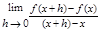(1)

The exponents of f(x+h), f(x), (x+h) and x are 1. The exponents of these terms can be considered as real number such as α. The general definition for derivative is given in Definition 1.

Definition 1: f(x):R→R is a function, αÎR and the Fractional Order Derivative (FOD) can be considered as follows.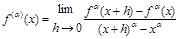(2)

In the case of very small value of h, the limit in the Definition 1 concluded in indefinite limit.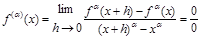(3)

In this case, the method used for indefinite limit (such as L’Hospital method) can be used, and the FOD can be redefined as in Definition 2.

Definition 2: Assume that f(x):R→R is a function, αÎR and L(.) be a L’Hospital process. The FOD of f(x) is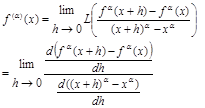(4)

3. Chain Rule and Proposed Method

Assume that u is a function, then the fractional order derivative of u isand αÎR. So, the chain rule for u must be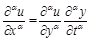(5)

There is not any definition for fractional order derivative in this way. He and his friends (2012) have definition for chain rule for fractional order derivative such as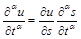(6)

where the first term in the right-hand side is a classical derivative of u with respect to s. He and his friends (2012) verified that the definitions of fractional order derivatives do not satisfy this equation. They have a counter-example for this case. This counter-example can be summarized as follow.

u(t)=t2, s(x)=xb, bÎR+.

The left-hand side of equation 6 is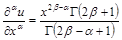(7)

The right-hand side of equation 6 is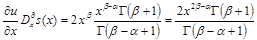(8)

It can be seen that equations 7 and 8 are not equal. He and his friends tried to satisfy this equation by multiplying right-hand side of equation 6 by a constant coefficient. Due to this case, the chain rule should be defined by using Definition 2.

Theorem 1: The chain rule for fractional order derivative with respect to Definition 2 is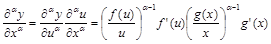(9)

where αÎR, y=f(u), and u=g(x).

Proof: y and u are not absolutely independent variables; however, x is an independent variable. The derivative means that the derivative is the response of dependent variable to changes in independent variables. So, the change in y with respect to u is not absolutely derivative, since u is not independent variable. The change in y with respect to x through u must be taken in care. So,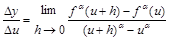and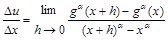.

The definition for fractional order derivative with respect to Definition 2 is as follow.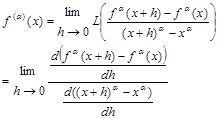The chain rule result is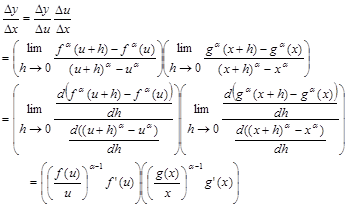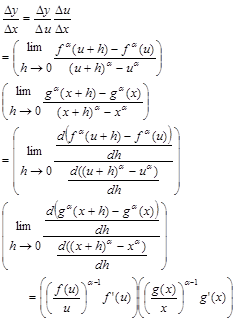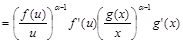.

Some examples can be given for sake of clarity of the chain rule and its application. Examples can be selected from polynomial, exponential, logarithmic and trigonometric functions.

Example 1: (Polynomial) Assume that y(x)=x2 and x(t)=2t+1. The left-hand side result and right-hand side result can be obtained separately.

LHS: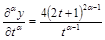and

RHS: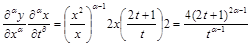It can be seen easily that LHS=RHS. When α=1, the result of definition of classical derivative is obtained. This means that Definition 2 for fractional order derivative is a complete and sound approach. When α=1, LHS=RHS=4(2t+1). The chain rule result for definition of classical derivative is (2x)2=4(2t+1).

Example 2: (Exponential) Assume that y(x)=ex and x(t)=2t+1. The left-hand side result and right-hand side result can be obtained separately.

LHS: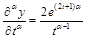and

RHS: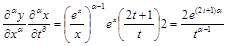It can be seen easily that LHS=RHS.

Example 3: (Trigonometric) Assume that y(x)=sin(x) and x(t)=t2. The left-hand side result and right-hand side result can be obtained separately.

LHS: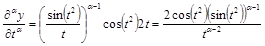and

RHS: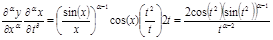It can be seen easily that LHS=RHS.

Example 4: (Logarithmic) Assume that y(x)=ln(x) and x(t)=2t. The left-hand side result and right-hand side result can be obtained separately.

LHS:and

RHS: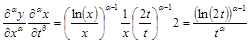It can be seen easily that LHS=RHS.

Theorem 2: Assume that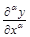and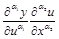derivatives, and α, α1, α2ÎR. Ifis left-hand side of chain rule andis right-hand side of chain rule, then α=α12.

Proof: The left-hand side is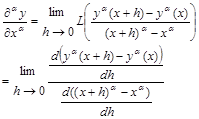and the terms in the right-hand side areand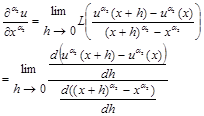In the case of chain rule,andmust be equal.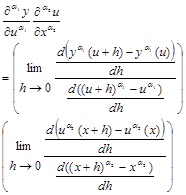In order to validate the chain rule,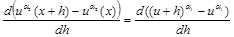.

This means that α12. Then the right-hand side will be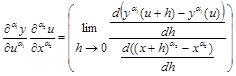.

If this is a chain rule, then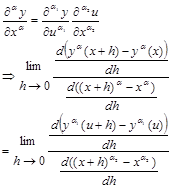The last equation verifies that all orders of derivative for chain rule must be equal.

Example 5: (Logarithmic) Assume that y(x)=ln(x) and x(t)=t2. The left-hand side result and right-hand side result can be obtained separately.

LHS:and

RHS: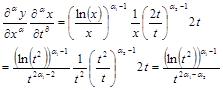In order to satisfy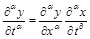, α-1=α1-1 and 2α12=α. It can be shown that α12=α.

4. Conclusions

In this paper, the methods for fractional order derivative are not valid chain rule based on study of He and his friends. Due to this case, a new definition for chain rule was developed in this paper. The developed method (definition) was verified by two theorems and the results of theorems were applied to polynomial, exponential, logarithmic and trigonometric functions. These examples illustrated that the proposed method is valid for fractional order derivative.

References

1. Newton, I.Philosophiæ Naturalis Principia Mathematica, 1687.
2. L'Hôpital, G. Analyse des Infiniment Petits pour l'Intelligence des Lignes Courbes ("Infinitesimal calculus with applications to curved lines"), Paris, 1696.
3. L'Hôpital, G. Analyse des infinement petits, Paris, 1715.
4. Baron, M.E. The Origin of the Infinitesimal Calculus, New York, 1969.
5. Leibniz, G.F. Correspondence with l‘Hospital, 1695.
6. Das, S. Functional Fractional Calculus, Springer-Verlag Berlin Heidelberg, 2011.
7. Mandelbrot, B.B., van Ness, J.W. Fractional Brownian motion, fractional noise and applications, SIAM Rev. 10: 422, 1968.
8. Mirevski, S.P., Boyadjiev, L., Scherer, R. On the Riemann-Liouville Fractional Calculus, g-Jacobi Functions and F.Gauss Functions, Applied Mathematics and Computation, 187:315-325, 2007.
9. Schiavone, S.E., Lamb, W. A Fractional Power Approach to Fractional Calculus, Journal of Mathematical Analysis and Applications, 149:377-401, 1990.
10. Bataineh, A.S., Alomari, A.K., Noorani, M.S.M., Hashim, I., Nazar, R. Series Solutions of Systems of Nonlinear Fractional Differential Equations, Acta Applied Mathematics, 105:189-198, 2009.
11. Diethelm, K., Ford, N.J., Freed, A.D., Luchko, Y. Algorithms fort he Fractional Calculus: A Selection of Numerical Methdos, Computer Methods in Applied Mechanics and Engineering, 194:743-773, 2005.
12. Li, C., Chen,A., Ye,J. Numerical Approaches to Fractional Calculus and Fractional Ordinary Differential Equation, Journal of Computational Physics, 230:3352-3368, 2011.
13. He, J.-H., Elagan,S.K., Li,Z.B.Geometrical explanation of the fractional complex transform and derivative chain rule for fractional calculus, Physics Letters A, 376:257-259, 2012.
14. Karcı, A.Kesirli Türev için Yapılan Tanımlamaların Eksiklikleri ve Yeni Yaklaşım, TOK-2013 Turkish Automatic Control National Meeting and Exhibition, Malatya, Turkey, 2013.
15. Karcı, A. A New Approach for Fractional Order Derivative and Its Applications, Universal Journal of Engineering Sciences, 1:110-117, 2013.
16. Karcı, A., Karadoğan, A. Fractional Order Derivative and Relationship between Derivative and Complex Functions, IECMSA-2013:2nd International Eurasian Conference on Mathematical Sciences and Applications,Sarajevo, Bosnia and Herzogovina, 2013.
17. Karcı, A., Karadoğan, A. Fractional Order Derivative and Relationship between Derivative and Complex Functions, Mathematical Sciences and Applications E-Notes, 2:44-54, 2014.

 Contents 1. 2. 3. 4.
Article ToolsAbstractPDF(166K)